# Endomorphism ring

The associative ring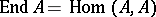consisting of all morphisms ofinto itself, whereis an object in some additive category. The multiplication in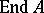is composition of morphisms, and addition is the addition of morphisms defined by the axioms of the additive category. The identity morphismis the unit element of the ring. An elementinis invertible if and only ifis an automorphism of the object. Ifandare objects of an additive category, then the grouphas the natural structure of a right module overand of a left module over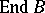. Letbe a covariant (or contravariant) additive functor from an additive categoryinto an additive category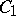. Then for any objectinthe functorinduces a natural homomorphism (or anti-homomorphism)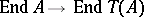.
Letbe the category of modules over a ring. For an-modulethe ringconsists of all endomorphisms of the Abelian groupthat commute with multiplication by elements of. The sum of two endomorphismandis defined by the formula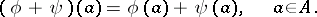Ifis commutative, thenhas the natural structure of an-algebra. Many properties of the modulecan be characterized in terms of. For example,is an irreducible module if and only ifis a skew-field.
An arbitrary homomorphismof an associative ringintois called a representation of the ring(by endomorphisms of the object). Ifhas a unit element, then one imposes the additional condition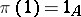. Any associative ringhas a faithful representation in the endomorphism ring of a certain Abelian group. If, moreover, has a unit element, thencan be chosen as the additive group ofon which the elements ofact by left multiplication. Ifhas no unit element andis obtained fromby adjoining a unit toexternally, thencan be taken to be the additive group of.
In the case of an Abelian varietyone considers, apart from the ring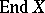, which is a finitely-generated-module, the algebra of endomorphisms (algebra of complex multiplications)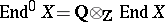.